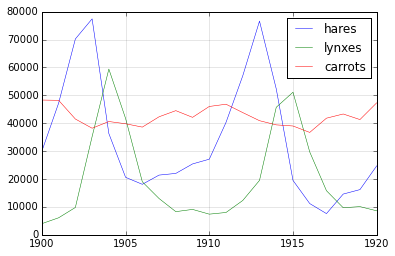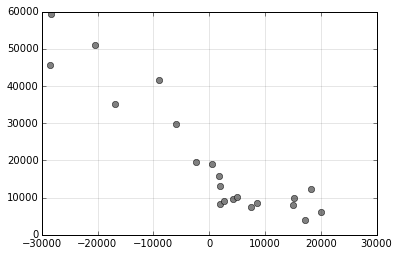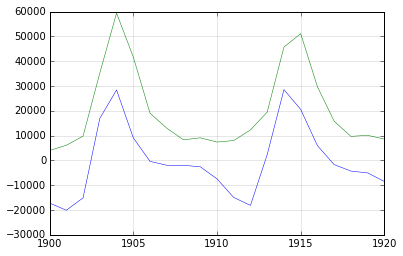In :
import numpy as np
import matplotlib.pyplot as plt

In :
%matplotlib inline


The population of Hares, Lynxes and carrots in Canada is in the file population.txt. Download that file and place it in this directory.

Once this is done, execute the code below to load the data in the variable data.

Important remark: you should not use any for loop in this exercise! :-)

In :
data = np.loadtxt('populations.txt')

In :
year, hares, lynxes, carrots = data.T  # trick: columns to variables

In :
year, hares, lynxes, carrots = data.T
populations = data[:,1:]

In :
data

Out:
array([[  1900.,  30000.,   4000.,  48300.],
[  1901.,  47200.,   6100.,  48200.],
[  1902.,  70200.,   9800.,  41500.],
[  1903.,  77400.,  35200.,  38200.],
[  1904.,  36300.,  59400.,  40600.],
[  1905.,  20600.,  41700.,  39800.],
[  1906.,  18100.,  19000.,  38600.],
[  1907.,  21400.,  13000.,  42300.],
[  1908.,  22000.,   8300.,  44500.],
[  1909.,  25400.,   9100.,  42100.],
[  1910.,  27100.,   7400.,  46000.],
[  1911.,  40300.,   8000.,  46800.],
[  1912.,  57000.,  12300.,  43800.],
[  1913.,  76600.,  19500.,  40900.],
[  1914.,  52300.,  45700.,  39400.],
[  1915.,  19500.,  51100.,  39000.],
[  1916.,  11200.,  29700.,  36700.],
[  1917.,   7600.,  15800.,  41800.],
[  1918.,  14600.,   9700.,  43300.],
[  1919.,  16200.,  10100.,  41300.],
[  1920.,  24700.,   8600.,  47300.]])

Plot the data (year vs each population item), using label and legend.

In :
for elt, name in zip(populations.T, ["hares", "lynxes", "carrots"]):
plt.plot(year, elt, label=name, lw=.5)
plt.legend()
plt.grid(ls='-', alpha=.2)Compute the mean (np.mean?) and standard deviation (np.std?) of each piece of data

In :
np.mean(data, axis=0)

Out:
array([  1910.        ,  34080.95238095,  20166.66666667,  42400.        ])
In :
np.std(data, axis=0)

Out:
array([  6.05530071e+00,   2.08979065e+04,   1.62545915e+04,
3.32250623e+03])

Which year each species had the largest population? (np.argmax? and use fancy indexing)

In :
year[np.argmax(data[:,1:], axis=0)]

Out:
array([ 1903.,  1904.,  1900.])

Which year any of the population is over 50000?

In :
mask = data[:,1:] > 50000

In :
year[np.any(mask, axis=1)]

Out:
array([ 1902.,  1903.,  1904.,  1912.,  1913.,  1914.,  1915.])

The top two years for each species when they had the lowest population

You may use argsort, which gives the indices for which the array value would be sorted.

In :
np.argsort?

In :
year[np.argsort(data[:,1:], axis=0)[:2]]

Out:
array([[ 1917.,  1900.,  1916.],
[ 1916.,  1901.,  1903.]])

Compare (plot) the change in hare population (see np.gradient?) and the number of lynxes.

In :
np.gradient(hares)

Out:
array([ 17200.,  20100.,  15100., -16950., -28400.,  -9100.,    400.,
1950.,   2000.,   2550.,   7450.,  14950.,  18150.,  -2350.,
-28550., -20550.,  -5950.,   1700.,   4300.,   5050.,   8500.])
In :
dhares = np.gradient(hares)
plt.plot(dhares, lynxes, 'o', mfc='gray', mec='black')
plt.grid(ls='-', alpha=.2)In :
plt.plot(year, -dhares, lw=.5)
plt.plot(year, lynxes, lw=.5)
plt.grid(ls='-', alpha=.2)In [ ]: